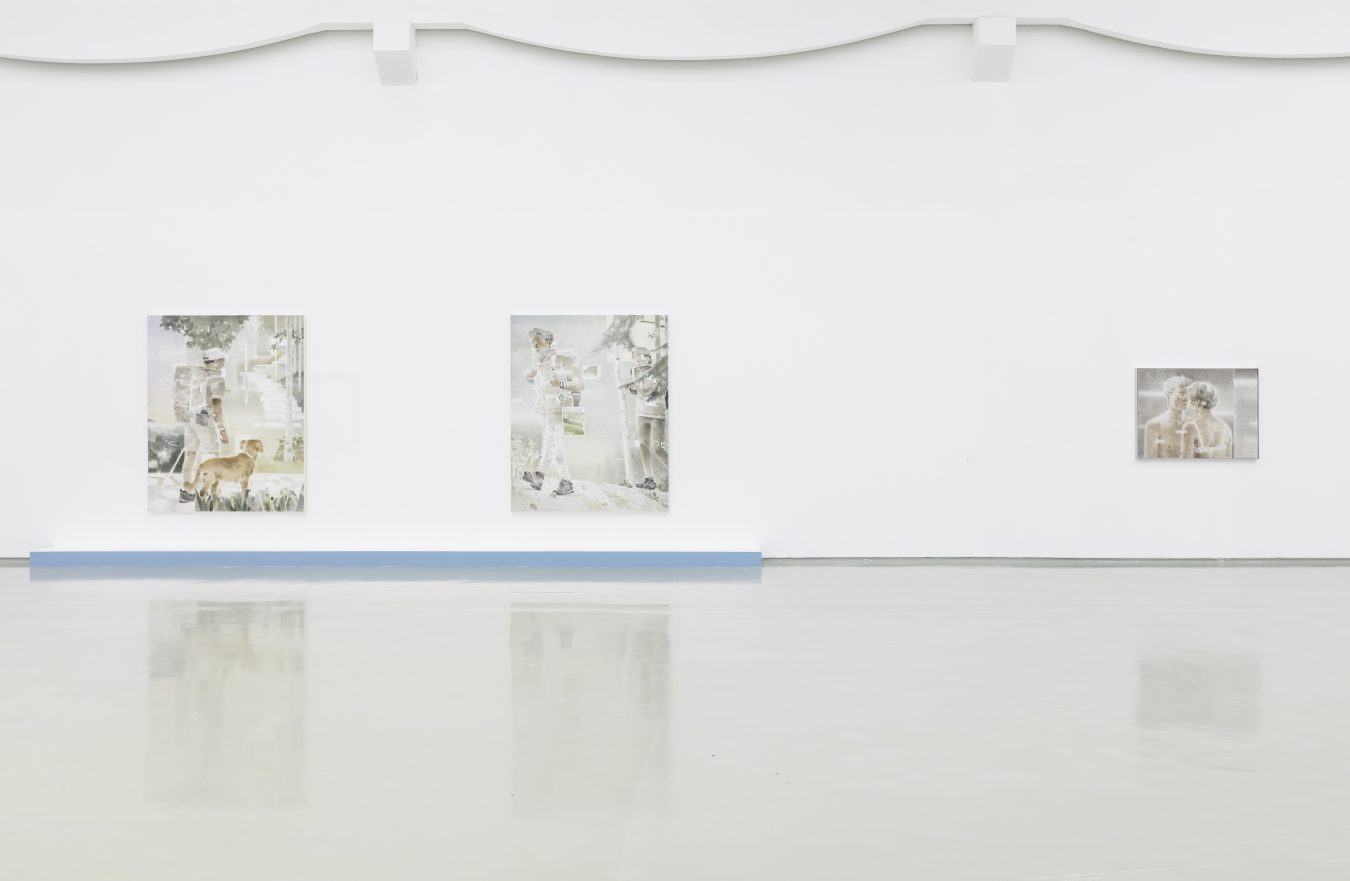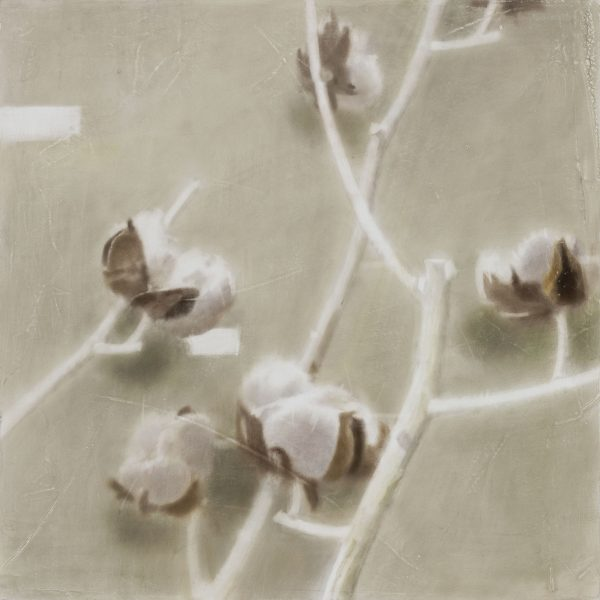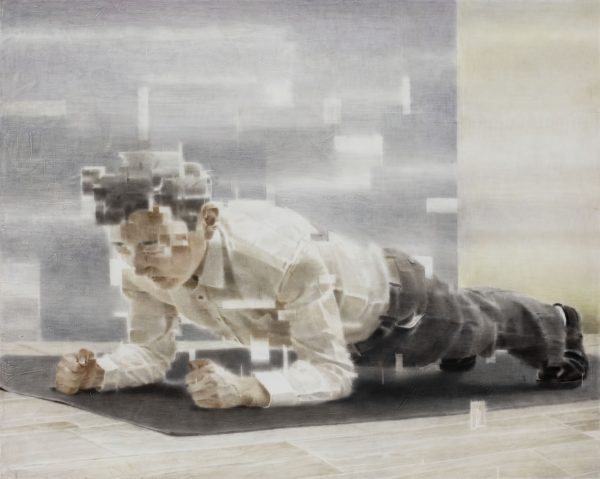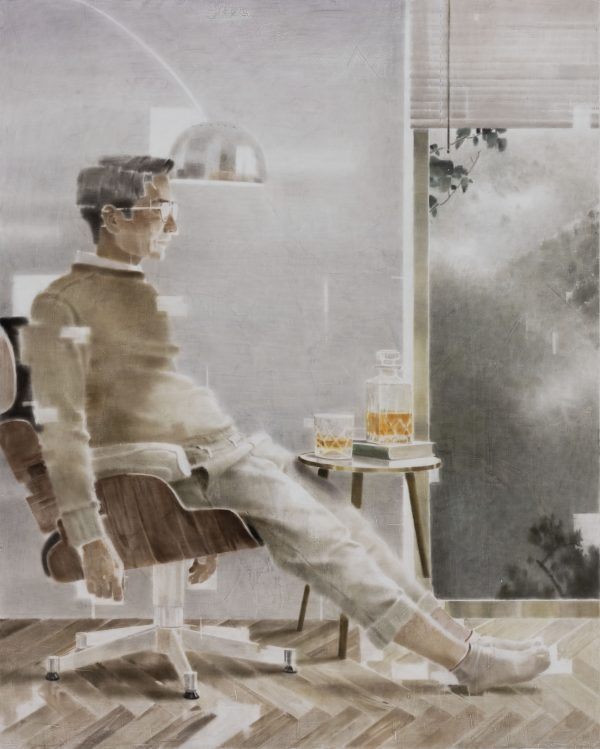2021. 木板坦培拉. 40×50cm.2021. 木板坦培拉. 40×40cm.2021. 木板坦培拉. 160×200cm.2021. 木板坦培拉. 200×160cm.Sober

2021. 木板坦培拉. 200×160cm.2021. 木板坦培拉. 50×40cm.2021. 木板坦培拉. 200×160cm.2021. 木板坦培拉. 200×160cm.2021. 木板坦培拉. 100×80cm.2021. 木板坦培拉. 200×160cm.2021. 木板坦培拉. 100×80cm.2021. 木板坦培拉. 120×90cm.2021. 木板坦培拉. 200×160cm.2021. 木板坦培拉. 40×40cm.